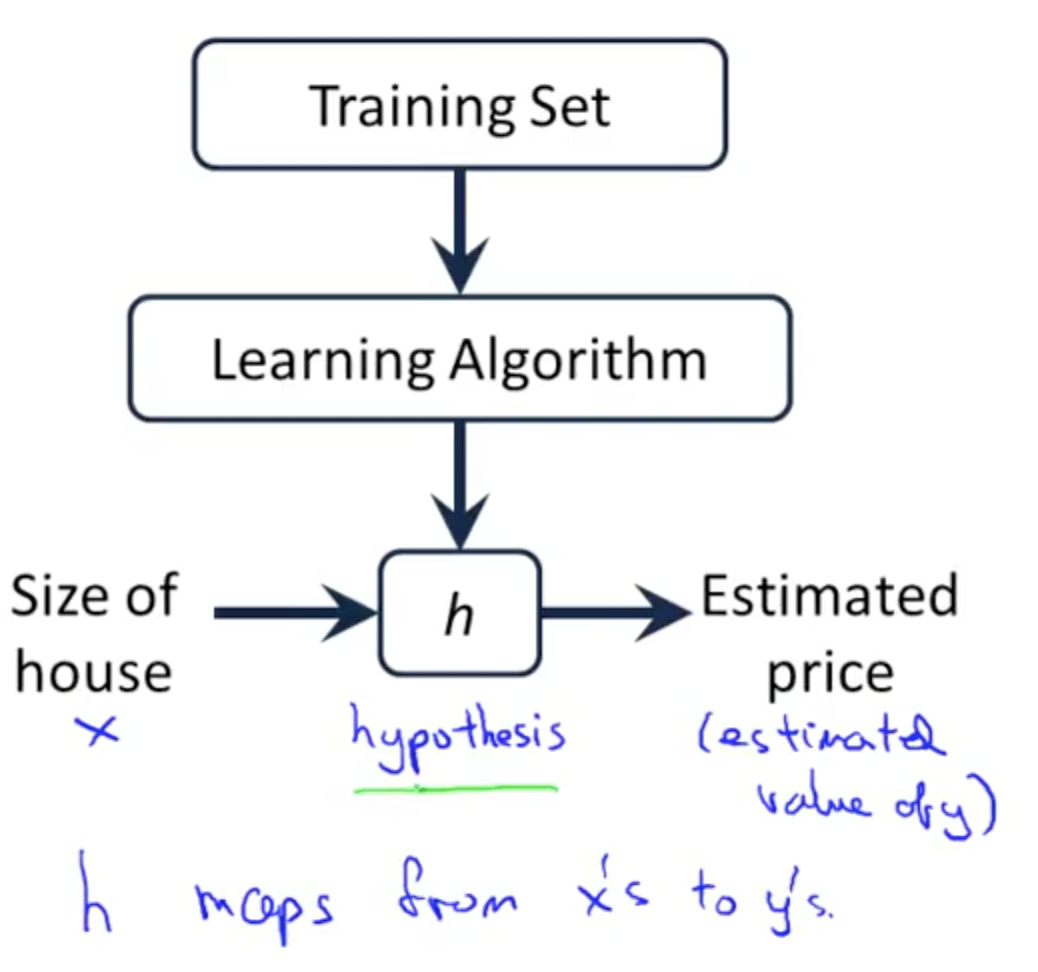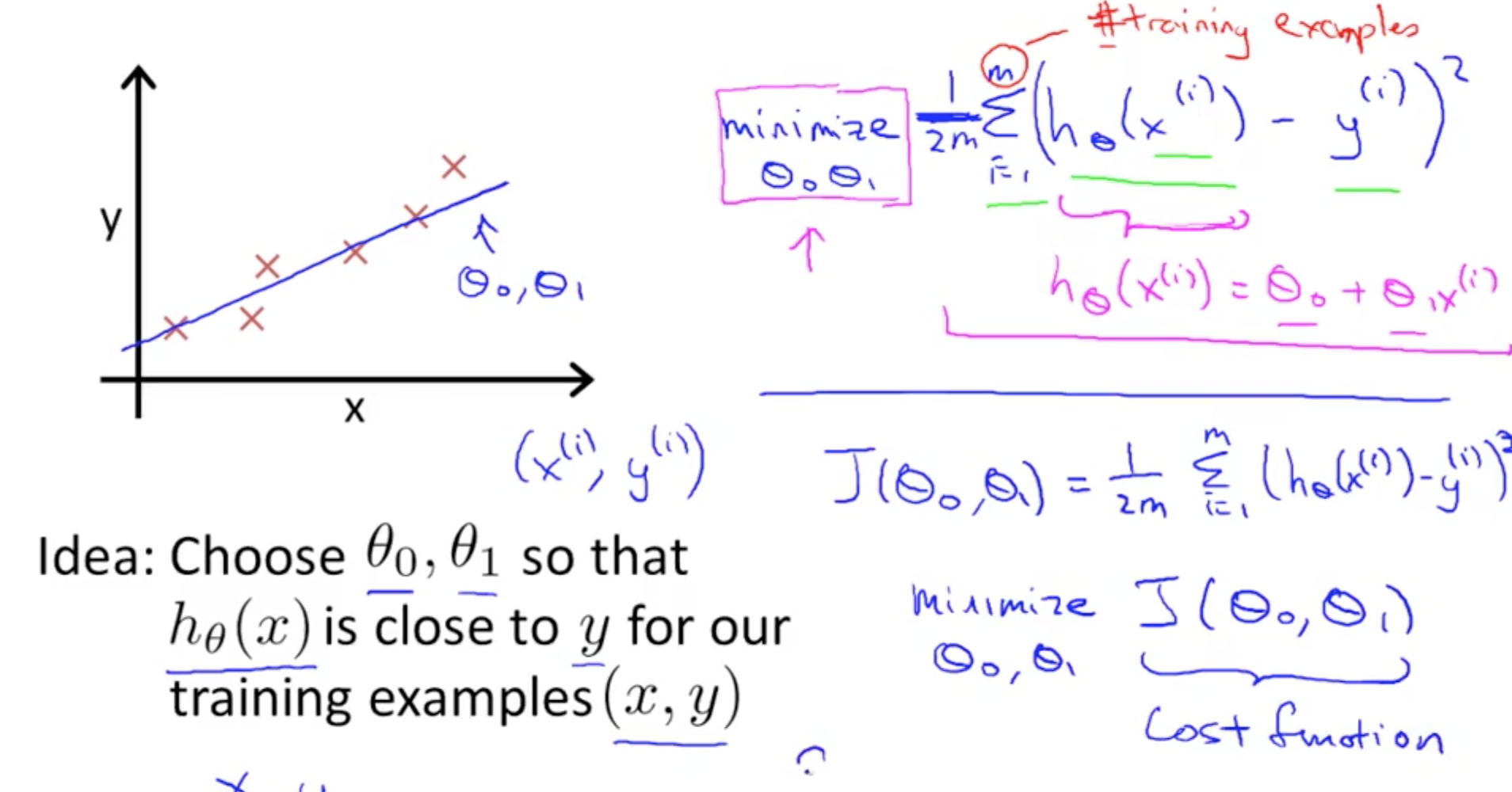# 機器學習 - model and cost function

### Model Representation : article

Housing Prices• Supervised Learning:
• Regression Problems• Classification Problems

Training set of housing prices

 Size in feet^2 (x) Price (\$) in 1000’s (y) 2104 460 1416 232 1534 315 … …
``````Notation:
m = Number of training examples
x's = "input" variable / features
y's = "output" variable / "target" variable
``````

Process of Hypothesis Function:When the target variable that we’re trying to predict is continuous, such as in our housing example, we call the learning problem a regression problem. When y can take on only a small number of discrete values (such as if, given the living area, we wanted to predict if a dwelling is a house or an apartment, say), we call it a classification problem.

### Cost Function : article

Cost function : will let us figure out how to fit the best possible straight line to our data

Linear Regression :

``````Hypothesis : hθ(x) = θ0 + θ1x
θi's : Parameters
Linear Regression Model:
J( θ0, θ1 ) =  1/2m * ∑ ( hθ * ( x^(i) ) - y^(i) )^2
``````Sometimes cost function is also called the squared error function

### Cost Function - Intuition i : article

• Goal : try to minimize the cost function J(θ0, θ1)``````Simplified: θ0 = 0
J(θ0, θ1) ---> J(θ1)
``````
``````when θ1 = 1
J(θ1) = 0
````````````when θ1 = 0.5
````````````when θ1 = 0
``````• We can find out in this case θ1 = 1 is our goal

``````when θ1 = 1
J(θ1) = 0
``````### Cost Fuction - Intuition ii : article• When we have 2 parameters the plot will be a 3D plot

``````EX:
θ0 = 50
θ1 = 0.06
hθ = 50 + 0.06x
``````
• J(θ0, θ1): 2 parameters• Contour graphs

• hθ(x) θ0 + θ1x• hθ(x) 360
``````θ0 = 360
θ1 = 0
``````• minimize the cost functionUpdated: